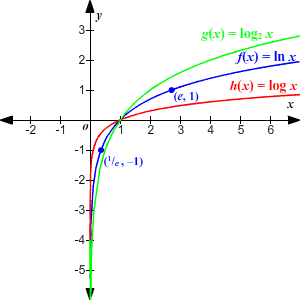# Natural Logarithm

The natural logarithm of a number $x$ is the logarithm to the base $e$ , where $e$ is the mathematical constant approximately equal to $2.718$ . It is usually written using the shorthand notation $\mathrm{ln}x$ , instead of ${\mathrm{log}}_{e}x$ as you might expect . You can rewrite a natural logarithm in exponential form as follows:

$\mathrm{ln}x=a⇔{e}^{a}=x$

Example 1:

Find $\mathrm{ln}7$ .

On a scientific calculator, you can simply press $\left[7\right]$ followed by $\left[\mathrm{ln}\right]$ to get the answer: approximately $1.946$ .

The exponential form of the equation you're solving is

$\begin{array}{l}{e}^{a}=7\\ {2.718}^{1.954}\approx 7\end{array}$

Example 2:

Solve the equation. Round to the nearest thousandth.

$\mathrm{ln}x=-5.5$

First, rewrite the equation in exponential form.

${e}^{-5.5}=x$

Use a calculator. (Most scientific calculators have a button which gives a good approximation for $e$ ; if yours doesn't have one, use $2.71828$ .)

$x\approx 0.004$

The usual properties of logarithms are also true for the natural logarithm.

Example 3:

Simplify.

$\mathrm{ln}{\left(3q\right)}^{2}$

The following property lets you simplify logarithms of a power:

${\mathrm{log}}_{b}{x}^{y}=y{\mathrm{log}}_{b}x$

So,

$\mathrm{ln}{\left(3q\right)}^{2}=2\mathrm{ln}\left(3q\right)$

Now use the property that the log of a product is equal to the sum of the logs.

${\mathrm{log}}_{b}xy={\mathrm{log}}_{b}x+{\mathrm{log}}_{b}y$

So,

$\begin{array}{l}2\mathrm{ln}\left(3q\right)=2\left(\mathrm{ln}3+\mathrm{ln}q\right)\\ \text{\hspace{0.17em}}\text{\hspace{0.17em}}\text{\hspace{0.17em}}\text{\hspace{0.17em}}\text{\hspace{0.17em}}\text{\hspace{0.17em}}\text{\hspace{0.17em}}\text{\hspace{0.17em}}\text{\hspace{0.17em}}\text{\hspace{0.17em}}\text{\hspace{0.17em}}\text{\hspace{0.17em}}\text{\hspace{0.17em}}\text{\hspace{0.17em}}\text{\hspace{0.17em}}\text{\hspace{0.17em}}\text{\hspace{0.17em}}\text{\hspace{0.17em}}\text{\hspace{0.17em}}\approx 2.197+2\mathrm{ln}q\end{array}$

The graph of the logarithmic function $f\left(x\right)=\mathrm{ln}x$ (shown in blue, below) looks similar to the graphs of related functions $g\left(x\right)={\mathrm{log}}_{2}x$ or $h\left(x\right)=\mathrm{log}x$ (remember that if no base is written, the base of the logairthm is understood to be $10$ ).

The function has an asymptote at $x=0$ and an $x$ -intercept at $\left(1,0\right)$ . It passes through the points $\left(\frac{1}{e},-1\right)$ and $\left(e,1\right)$ .